## Introduction to Depreciation

Buildings, machinery, equipment, furniture, fixtures, computers, outdoor lighting, parking lots, cars, and trucks are examples of assets that will last for more than one year, but will not last indefinitely. During each accounting period (year, quarter, month, etc.) a portion of the cost of these assets is being used up. The portion being used up is reported as Depreciation Expense on the income statement. In effect depreciation is the transfer of a portion of the asset's cost from the balance sheet to the income statement during each year of the asset's life.
The calculation and reporting of depreciation is based upon two accounting principles:
1. Cost principle. This principle requires that the Depreciation Expense reported on the income statement, and the asset amount that is reported on the balance sheet, should be based on the historical (original) cost of the asset. (The amounts should not be based on the cost to replace the asset, or on the current market value of the asset, etc.)
2. Matching principle. This principle requires that the asset's cost be allocated to Depreciation Expense over the life of the asset. In effect the cost of the asset is divided up with some of the cost being reported on each of the income statements issued during the life of the asset. By assigning a portion of the asset's cost to various income statements, the accountant is matching a portion of the asset's cost with each period in which the asset is used. Hopefully this also means that the asset's cost is being matched with the revenues earned by using the asset.
There are several depreciation methods allowed for achieving the matching principle. The depreciation methods can be grouped into two categories: straight-line depreciation and accelerated depreciation.
The assets mentioned above are often referred to as fixed assets, plant assets, depreciable assets, constructed assets, and property, plant and equipment. It is important to note that the asset is not depreciated, because land is assumed to last indefinitely.
Note: We developed forms and exam questions to help you learn about depreciation. They are available at

## Book vs. Tax Depreciation

AccountingCoach.com's discussion of depreciation is limited to the depreciation entered into the company's (or books) and reported on the company's financial statements. These amounts are based on accounting principles. The amounts resulting from the accounting principles are often different from the amounts based on the Internal Revenue Service code and regulations. Hence the depreciation on the financial statements will likely be legitimately different from the depreciation on the company's tax returns. [To learn about the depreciation for income tax purposes, you should review the Internal Revenue Service publications (available via the Internet) and/or consult a tax professional.]

## Book Depreciation Illustrated

### Assumptions

To illustrate depreciation used in the accounting records and on the financial statements, let's assume the following facts:
• On July 1, 2012 a company purchases equipment having a cost of \$10,500.
• The company estimates that the equipment will have a useful life of 5 years.
• At the end of its useful life, the company expects to sell the equipment for \$500.
• The company wants the depreciation to be reported evenly over the 5-year life.

### Calculation of Straight-line Depreciation

The most common method of depreciating assets for financial statement purposes (as opposed to the method used for income tax purposes) is the straight-line method. Under this depreciation method, the depreciation for each full year is the same amount.
The depreciation expense for a full year when computed under the straight-line method is illustrated here:If a company's accounting year ends on December 31, the company will report the depreciation expense on the company's income statement as shown in the following depreciation schedule: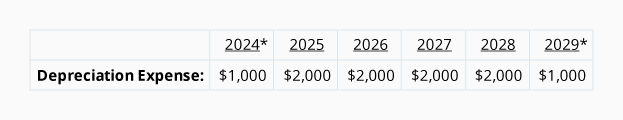The actual cash paid by the company for this equipment will occur as follows: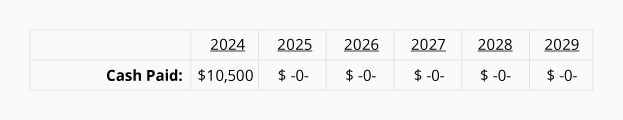As you can see, the company paid \$10,500 in 2012, but the 2012 income statement reports Depreciation Expense of only \$1,000. (Because the asset was acquired on July 1, 2012, only half of the annual depreciation expense amount is recorded in 2012 and 2017.) In each of the years 2013 through 2016 the company's income statements will report \$2,000 of Depreciation Expense, thereby matching \$2,000 of Depreciation Expense with the revenues earned in each of those years. However, the company will not pay out any cash for this expense during those years. The company's before income taxes will be reduced in each of the years 2013 through 2016 by \$2,000â€”but the Cash account will not be reduced. This explains why Depreciation Expense is sometimes referred to as a noncash expense.

## Journal Entries For Depreciation

The depreciation for the financial statements is entered into the accounts via a  Assuming that the company prepares only annual financial statements the journal entries can be prepared as of the last day of each year: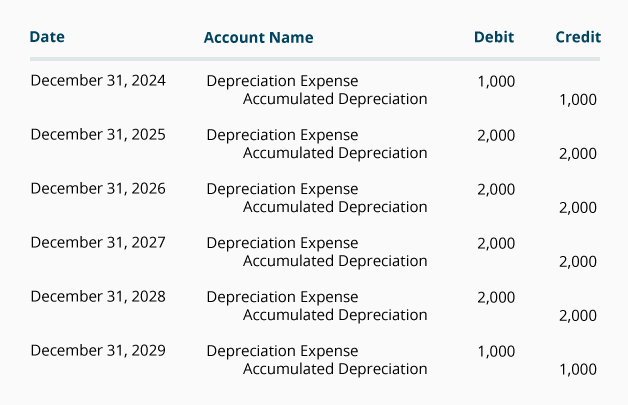If monthly financial statements were prepared, 1/12 of the annual amounts would be entered monthly.
Note that the account credited in the journal entries is not the asset account  Instead, the credit is entered in the Accumulated Depreciation. The use of this contra account will allow the asset Equipment to continue to report the equipment's cost, while also reporting in the account Accumulated Depreciation the amount that has been charged to Depreciation Expense since the asset was acquired. For example, as of December 31, 2013 the Equipment account will have a debit balance of \$10,500. On the same day, the account Accumulated Depreciation will have a credit balance of \$3,000. In T-account form, it looks like this: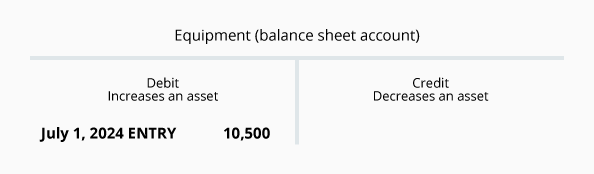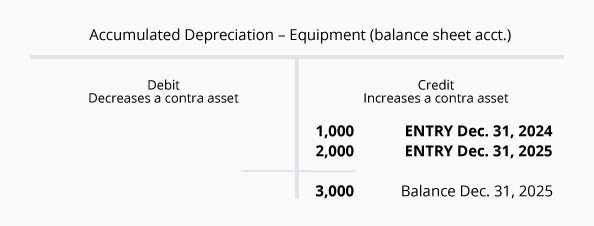The \$10,500 debit balance in Equipment minus the \$3,000 credit balance in Accumulated Depreciation equals \$7,500. This amount of \$7,500 is referred to as the or as the of the equipment.

## Use of Estimates

### Examples of Estimates

The calculation of depreciation (shown at the end of Part 1) included two estimates:
1. Salvage value. Salvage value is the estimated amount that a company will receive when it disposes of an asset at the end of the asset's useful life. Often the salvage value is estimated to be zero. However, we assumed \$500 in order to demonstrate how an amount would be handled. Salvage value is also referred to as disposal value, scrap value, or residual value.
2. Useful life. The useful life of an asset is an estimate of how long the asset will be used (as opposed to how long the asset will last). For example, a graphic artist might purchase a computer in 2012 and expects to replace it in 2014 with a more advanced computer. Hence the graphic artist's computer will have an estimated useful life of 2 years. An accountant purchasing a similar computer in 2012 expects to use it until 2016. The accountant will use an estimated useful life of 4 years when computing depreciation. Both the graphic artist and the accountant are correct—the graphic artist in using 2 years and the accountant in using 4 years—even if the computers will be in working order for many years after their useful lives end.

### Changes in Estimates

Whenever estimates are used in accounting, it is possible they will change as time moves forward. For example, a company bought a machine for \$14,000 on January 1, 2008. At the time it was estimated to have no salvage value at the end of its useful life estimated to be 7 years. The company used straight-line depreciation. In 2012 the company realizes that technology will cause the machine to be obsolete by December 31, 2013 and there will be no salvage value at that time. Instead of the original useful life of 7 years, the company now estimates a total useful life of only 6 years (January 1, 2008 through December 31, 2013). This change in the estimated useful life affects only the current and future years. In other words, in this example the depreciation for 2012 and 2013 will be affected. The depreciation already reported for the years 2008, 2009, 2010, and 2011 cannot be changed. Any amount not depreciated as of December 31, 2011 will have to be depreciated over the years 2012 and 2013.
Let's first calculate the straight-line depreciation using the estimates in January 2008:
In the T-accounts we can see the cost of the Equipment \$14,000 and the Accumulated Depreciation of \$8,000 as of December 31, 2011:
These accounts show that \$6,000 (\$14,000 - \$8,000) remains on the books at December 31, 2011 and there are only two years remaining (2012 and 2013) in which to depreciate the remaining \$6,000. The remaining \$6,000 will be divided by the 2 years remaining and will result in \$3,000 of depreciation in each of the years 2012 and 2013.
In general journal format the entries will be:
At the end of 2013 the Accumulated Depreciation account will look like this:
Note that the depreciation amounts recorded in the years 2011 and before were not changed.

## Accelerated Depreciation

### What Is It?

Accelerated depreciation is an alternative to the method. Compared to the straight-line method, accelerated depreciation methods provide for more depreciation in the early years of an asset's life but then less depreciation in the later years. Under any depreciation method, the maximum depreciation during the life of an asset is limited to the cost of the asset. The difference in depreciation methods involves when you will report the depreciation. It's a matter of timing. Again, the total depreciation during the life of the asset is the same regardless of the depreciation method used.
As stated earlier, most companies use the straight-line method of depreciation for their financial statements. It is easy to compute and to understand. With straight-line depreciation the company will have the same amount of depreciation in each of the years of the asset's life. Accelerated depreciation will mean larger Depreciation Expense in the early years of the asset's life and then smaller Depreciation Expense in the later years. This larger expense in the earlier years will mean the company will report less profits in the earlier years of an asset's life (and greater profits in later years). Generally this is not appealing to most companies. As a result most companies will opt for the straight-line depreciation for their financial statements.
However, using an accelerated depreciation method on the company's income tax returns is very appealing. Higher depreciation in the early years of the asset means immediate income tax savings. Smaller depreciation in later years is far into the future. Generally, it is better to take the income tax savings sooner rather than later.
Fortunately a company is permitted to use straight-line depreciation on its financial statements and at the same time it can use accelerated depreciation on its income tax returns.

### Various Accelerated Depreciation Methods

There are various methods of accelerated depreciation. Here are some of them:
• Double-declining balance (also known as the 200% declining balance)
• 150% declining balance
• 125% declining balance
• Sum-of-the-years' digits
To learn more about these accelerated depreciation methods, refer to an Intermediate Accounting textbook. If you wish to use accelerated depreciation on your income tax return, refer to the Internal Revenue Service publications and/or consult with a tax professional.

#### Additional Information and Resources

Because the material covered here is considered an introduction to this topic, many complexities have been omitted. You should always consult with an accounting professional for assistance with your own specific circumstances.